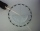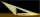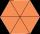# Ratio + angle - math problems

On solving problems and tasks with proportionally, we recommend hint rule of three. Rule of three (proportionality) help solve examples of direct and inverse proportionality. Three members make possible to calculate the fourth - unknown member.

#### Number of problems found: 49

• Powerplant chimneyFrom the window of the building at a height of 7.5 m, the top of the factory chimney can be seen at an altitude angle of 76° 30 ′. The base of the chimney can be seen from the same place at a depth angle of 5° 50 ′. How tall is the chimney?
• Sphere in coneA sphere is inscribed in the cone (the intersection of their boundaries consists of a circle and one point). The ratio of the surface of the ball and the contents of the base is 4: 3. A plane passing through the axis of a cone cuts the cone in an isoscele
• Interior anglesCalculate the interior angles of a triangle that are in the ratio 2: 3: 4.
• Refractive indexThe light passes through the interface between air and glass with a refractive index of 1.5. Find: (a) the angle of refraction if light strikes the interface from the air at an angle of 40°. (b) the angle of refraction when light strikes the glass interfa
• The angles ratioThe angles in the ABC triangle are in the ratio 1: 2: 3. find the sizes of the angles and determine what kind of a triangle it is.
• The towerThe observer sees the base of the tower 96 meters high at a depth of 30 degrees and 10 minutes and the top of the tower at a depth of 20 degrees and 50 minutes. How high is the observer above the horizontal plane on which the tower stands?
• Two bodiesThe rectangle with dimensions 8 cm and 4 cm is rotated 360º first around the longer side to form the first body. Then, we similarly rotate the rectangle around the shorter side b to form a second body. Determine the ratio of surfaces of the first and seco
• Cone sideCalculate the volume and area of the cone whose height is 10 cm and the axial section of the cone has an angle of 30 degrees between height and the cone side.
• The aspect ratioThe aspect ratio of the rectangular triangle is 13: 12: 5. Calculate the internal angles of the triangle.
• A triangleA triangle has an angle that is 63.1 other 2 are in ratio of 2:5 What are the measurements of the two angles?
• LighthouseMarcel (point J) lies in the grass and sees the top of the tent (point T) and behind it the top of the lighthouse (P). | TT '| = 1.2m, | PP '| = 36m, | JT '| = 5m. Marcel lies 15 meters away from the sea (M). Calculate the lighthouse distance from the sea
• Trapezium diagonalsIt is given trapezium ABCD with bases | AB | = 12 cm, |CD| = 8 cm. Point S is the intersection of the diagonals for which |AS| is 6 cm long. Calculate the length of the full diagonal AC.
• AnglesIn the triangle ABC, the ratio of angles is: a:b = 4: 5. The angle c is 36°. How big are the angles a, b?
• Isosceles triangle 8If the rate of the sides an isosceles triangle is 7:6:7, find the base angle correct to the nearest degree.A meter pole perpendicular to the ground throws a shadow of 40 cm long, the house throws a shadow 6 meters long. What is the height of the house?Tree perpendicular to the horizontal surface has a shadow 8.32 meters long. At the same time meter rod perpendicular to the horizontal surface has shadow 64 cm long. How tall is tree?
• The perimeterThe perimeter of equilateral △PQR is 12. The perimeter of regular hexagon STUVWX is also 12. What is the ratio of the area of △PQR to the area of STUVWX?
• Sun raysIf the sun's rays are at an angle 60° then famous Great Pyramid of Egypt (which is now high 137.3 meters) has 79.3 m long shadow. Calculate current height of neighboring chefren pyramid whose shadow is measured at the same time 78.8 m and the current heig
• Ratio iso triangleThe ratio of the sides of an isosceles triangle is 7:6:7 Find the base angle to the nearest answer correct to 3 significant figure.
• SSA and geometryThe distance between the points P and Q was 356 m measured in the terrain. The PQ line can be seen from the viewer at a viewing angle of 107° 22 '. The observer's distance from P is 271 m. Determine the viewing angle of P and observer.

Do you have an interesting mathematical word problem that you can't solve it? Submit a math problem, and we can try to solve it.

We will send a solution to your e-mail address. Solved examples are also published here. Please enter the e-mail correctly and check whether you don't have a full mailbox.

Please do not submit problems from current active competitions such as Mathematical Olympiad, correspondence seminars etc...

Check out our ratio calculator.